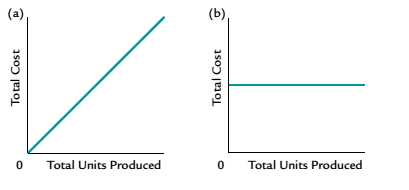# Which of the following graphs illustrates how total fixed costs be have with changes in total units produced### Survey of Accounting (Accounting I)

8th Edition
Carl Warren
Publisher: Cengage Learning
ISBN: 9781305961883### Survey of Accounting (Accounting I)

8th Edition
Carl Warren
Publisher: Cengage Learning
ISBN: 9781305961883

#### Solutions

Chapter
Section
Chapter 11, Problem 6CDQ
Textbook Problem

## Which of the following graphs illustrates how total fixed costs be have with changes in total units producedExpert Solution
To determine

Concept Introduction:

Variable, Fixed and Mixed Cost:

There are three types of costs according to the unit of production; Variable, Fixed and Mixed. Variable costs change proportionally with the number of units produced and variable cost per unit remains constant. Fixed Cost remains same in totality irrespective of the number of units produced. The mixed cost is the mix of variable and fixed cost, some of its part is fixed and some variable.

To Indicate:

The behavior of Total fixed costs with change in total units produced

### Explanation of Solution

There are three types of costs according to the unit of production; Variable, Fixed and Mixed. Variable costs change proportionally with the number of units produced and variable cost per unit remains constant. Fixed Cost remains same in totality irrespective of the number of units produced...

### Want to see the full answer?

Check out a sample textbook solution.See solution

### Want to see this answer and more?

Bartleby provides explanations to thousands of textbook problems written by our experts, many with advanced degrees!

See solution